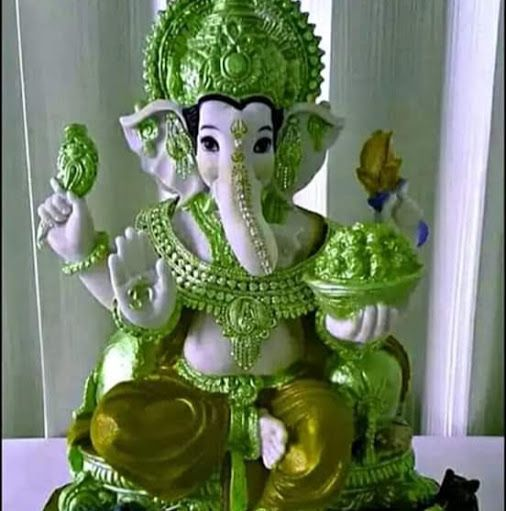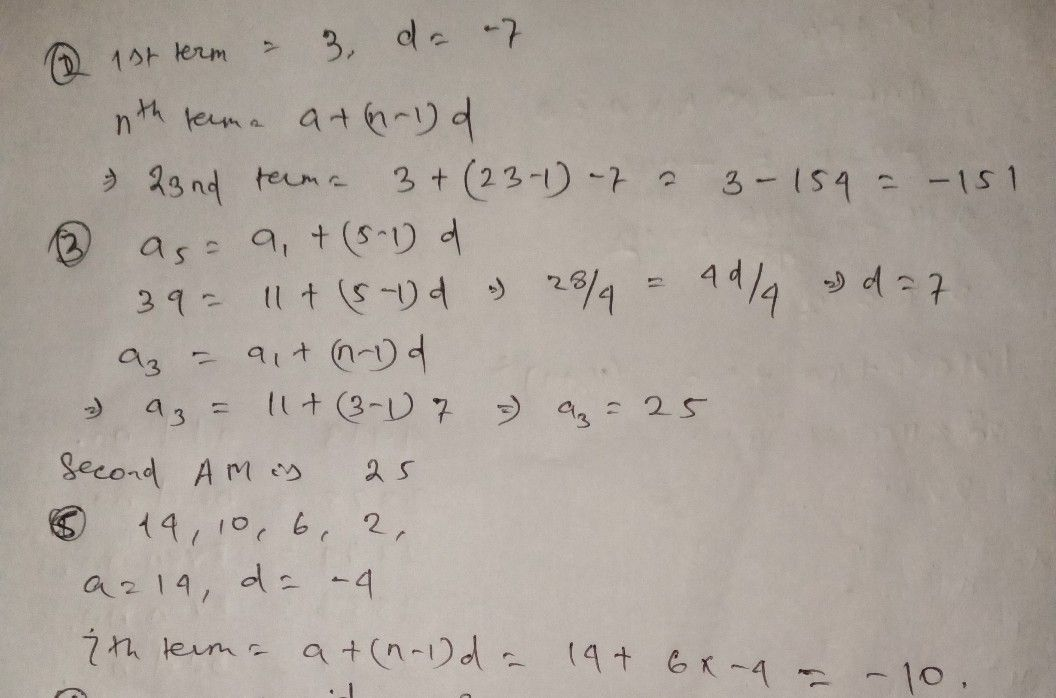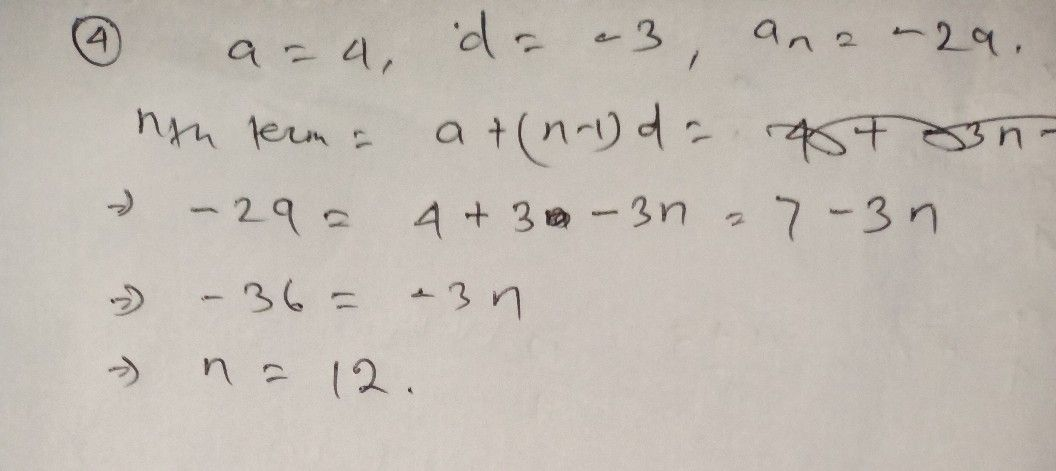Symbol
Problem2. The first term of an arithmetic sequence is 3 and the common difference $1s-7$ Find the $23d$ term. $A.-151$ B. $=144$ C. $=137$ D. $=130$ 3. If three arithmetic means are inserted between 11 and $39$ find the $seconG$ arithmetic mean. A. 18 B. 25 C. 32 D. 46 4. Which term of the arithmetic sequence 4, 1, $-2-5$ ... $1s-297$ A. 9th term B. 10th term C. $111n$ term D. 12th term $5.$ What is the 7h term of the arithmetic sequence $14$ $10.6.2$ $24.....2$ A. $+4$ B. - 5 $C.=$ -6 D. $=10$
10th-13th grade
Other
Search count: 120
SolutionQanda teacher - Suryakanta